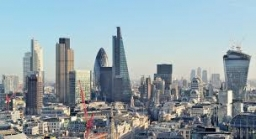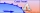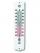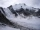# Equal temperature

The temperatures of the two cities were measured at the same time. The temperature in city A was 60 degrees And rose at a constant rate of 2 degrees per hour.

The temperature in city B was 40° and rose at a constant rate of 10° per hour
Enter the time in hours when the temperature is the same in both cities

t =  2.5 h

### Step-by-step explanation:

60 + 2t = 40+10t

60 + 2•t = 40+10•t

8t = 20

t = 5/2 = 2.5

Our simple equation calculator calculates it.Did you find an error or inaccuracy? Feel free to write us. Thank you!Tips to related online calculators
Do you have a linear equation or system of equations and looking for its solution? Or do you have a quadratic equation?
Do you want to convert velocity (speed) units?
Do you want to convert time units like minutes to seconds?

## Related math problems and questions:

• The temperature 4The temperature was very cold, then it doubled, then it dropped 10 degrees, and then it increased by 40 degrees. The temperature is now 16 degrees. What was the starting temperature?
• Temperature differenceLibya's highest temperature was recorded at 58 degrees Celsius, and the lowest was recorded at -88 degrees Celsius. What is the temperature difference?
• 3-bracket 3Two angles in a triangle are 90° and 60°. Has triangle at least two equal sides?
• The temperature 6The temperature was 47°F on Thursday and 60°F on Friday. How much did the temperature rise?
• TrianglesFind out whether given sizes of the angles can be interior angles of a triangle: a) 23°10',84°30',72°20' b) 90°,41°33',48°37' c) 14°51',90°,75°49' d) 58°58',59°59',60°3'
• OpheliaOphelia recorded the temperature of a cold store every two hours. 1. At 6 am, it was -4°C, and at 8 am, it was -1°C. By how much did the temperature rise? 2. The temperature went up by 5°C in the next two hours. .What was the temperature at 10 am?
• Train from BrnoFirst train started from Brno at 8 am at 60 km per hour. Second train started from Opava at 7 o'clock at the speed of 40 kilometers per hour. In how many hours and how far from cities trains meet when the distance of cities is 200 kilometers.
• A cold frontAt 1:00 PM, the temperature was at 46 degrees. Then, a cold front moved in and decreased the temperature 12 degrees per hour. The temperature at 6:00 PM = _____ degrees.
• The temp rangeWhat is the range in temperatures if the high temperatures is 67 degrees Celsius and the low temperature is -25 degrees Celsius?
• If the 3If the temperature of a city drops 2° everyday and increases by 3° on every 5 days, how long does it take to drop to -15° from temperature +5°?
• The bathDad poured 50 liters of water at 60°C into the tub. How much must cold water be added at 10°C to obtain a bath that has a temperature of 40°C?
• Outside temperatureThe temperature outside was 57 degree Fahrenheit. During the next few hours it decreased by 18 degrees and then increased by 23 degrees. Find new temperature.
• Temperature variationsToday's temperature was 80 degrees, and then the temperature dropped 10 degrees. Then it dropped 15 degrees again, then the next day, the temperature went up 2 degrees. What would the temperature be?
• Daily temperatureThe average of daily temperature measurements in one week every day at the same hour was -2.8 °C. We measured all temperatures on different days are different. The highest daily maximum temperature was 2.4 °C, the lowest -6 °C. Determine the options that
• The temperature 5The temperature at 4pm was 53° Fahrenheit the temperature dropped 3° each hour from 4pm to 9pm which equation below accurately represents the temperature at 9pm
• Cars 6At 9:00 am two cars started from the same town and traveled at a rate of 35 miles per hour and the other car traveled at a rate of 40 miles per hour. After how many hours will the cars be 30 miles apart?
• HandsThe clock shows 12 hours. After how many minutes will angle between the hour and minute hand 90°? Consider the continuous movement of both hands hours.